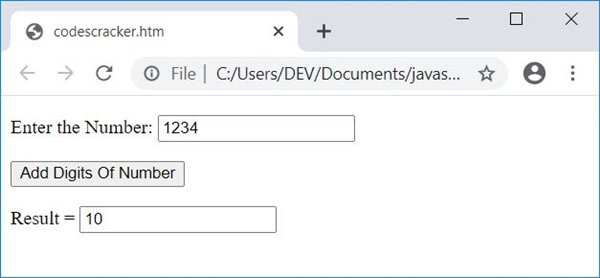# JavaScript Program to Add Digits of a Number

In this article, you will learn how to add the digits of a number in JavaScript. Here is the list of JavaScript programs you will go through:

## Add the digits of a number in JavaScript without user input

This program does not allow the user to enter the data. That is, this program only adds all the digits of a number, say 1234, and prints its addition result on output.

This is just a simple JavaScript code that shows you how the addition of all digits of a number gets performed.

```<!doctype html>
<html>
<body>
<script>
var num=1234, rem, sum=0;
while(num)
{
rem = num%10;
sum = sum+rem;
num = Math.floor(num/10);
}
document.write(sum);
</script>
</body>
</html>```

Save this code in a file with .html extension and open it in a web browser. Here is the output:Note: The Math.floor() method rounds the number to its nearest integer. That is, Math.floor(12/10) gives you 1 instead of 1.2.

The following code:

```while(num)
{
rem = num%10;
sum = sum+rem;
num = Math.floor(num/10);
}```

works in a way that:

• The condition of the while loop evaluates to true because the value of num is not 0, initially.
• So program flow goes inside the loop.
• There, num%10 or 1234%10 or 4 gets initialized to rem. So rem=4
• And sum+rem or 0+4 or 4 gets initialized to sum. So sum=4
• The final statement, Math.floor(num/10) or Math.floor(1234/10) or Math.floor(123.4) or 123, gets initialized to num.
• Now again, the condition of the while loop gets evaluated. Because the value of num is 123, which is not equal to 0, the condition evaluates to true again.
• So program flow again goes inside the loop and evaluates all three statements.
• This process continues until the condition of the while loop evaluates to be false.

The document.write() method writes the value of sum into an HTML output.

## Add the digits of a given number in JavaScript

This program allows the user to enter the number, then find and print the sum of the digits of that entered (given) number, as shown here:

```<!doctype html>
<html>
<script>
{
var num, rem, sum=0;
num = parseInt(document.getElementById("num").value);
while(num)
{
rem = num%10;
sum = sum+rem;
num = Math.floor(num/10);
}
document.getElementById("result").value = sum;
}
</script>
<body>

<p>Enter the Number: <input id="num"></p>
<p>Result = <input id="result"></p>

</body>
</html>```

Here is its sample output with user input, 1234:#### Live Output of the Previous Program

Here is the live output of the above JavaScript program on the addition of digits of a given number by the user:

Enter the Number:

Result =

After clicking on the button named Add Digits of Number, a function named addDigitsOfNumber() gets called. Therefore, all the statements inside this function get executed, and the value of input, whose id is result, gets changed with the value of sum.

JavaScript Online Test

« Previous Program Next Program »